# Can You Pass This Fourth Grade Math Test?

## Introduction

What do you think of when you hear "school"? Seeing your friends every day, discovering new fields of knowledge, or the feeling of dread when you realized you forgot about studying for that day's quiz? Some might remember math class. In fourth grade, teachers introduced us to more complicated concepts, such as multi-digit multiplication and division. That eternal question, "Am I ever going to use this in real life?", was certainly on many minds when preparing for an upcoming test on fraction equivalence. Little did we know there would be a time when all of this knowledge would come in handy!

Without this knowledge base, we wouldn't know how much that cute \$19.99 shirt will cost us after adding that amazing 70% discount, or how much to tip on our restaurant bills when we eat out. The concepts we learned in fourth grade certainly come in handy, whether or not we had fun learning them at the time.

U.S. Common Core now dictates that teachers should focus on the following criteria: multi-digit multiplications and divisions involving multi-digit dividends; understanding fraction equivalence, adding and subtracting like-denominator fractions, and multiplying fractions by whole numbers; and understanding some basic concepts regarding geometric figures (like symmetry). Common Core also encourages knowing basic measurements and their relative sizes within a system, even suggesting some knowledge of not just Imperial units (e.g., inches or feet), but also some basic metric units (e.g., cm or m) as well.

How much do you remember from fourth grade? Can you tell apart an isosceles from a scalene triangle? Do you know whether 4/8 is bigger than 2/4, or if they're equal to each other? If these questions rang a bell, take our quiz to see if you could pass a fourth grade U.S. math test! Click "Let's Play!" to begin!

## Description

What do you think of when you hear "school"? Seeing your friends every day, discovering new fields of knowledge, or the feeling of dread when you realized you forgot about studying for that day's quiz? Some might remember math class. In fourth grade, teachers introduced us to more complicated concepts, such as multi-digit multiplication and division. That eternal question, "Am I ever going to use this in real life?", was certainly on many minds when preparing for an upcoming test on fraction equivalence. Little did we know there would be a time when all of this knowledge would come in handy!

Without this knowledge base, we wouldn't know how much that cute \$19.99 shirt will cost us after adding that amazing 70% discount, or how much to tip on our restaurant bills when we eat out. The concepts we learned in fourth grade certainly come in handy, whether or not we had fun learning them at the time.

U.S. Common Core now dictates that teachers should focus on the following criteria: multi-digit multiplications and divisions involving multi-digit dividends; understanding fraction equivalence, adding and subtracting like-denominator fractions, and multiplying fractions by whole numbers; and understanding some basic concepts regarding geometric figures (like symmetry). Common Core also encourages knowing basic measurements and their relative sizes within a system, even suggesting some knowledge of not just Imperial units (e.g., inches or feet), but also some basic metric units (e.g., cm or m) as well.

How much do you remember from fourth grade? Can you tell apart an isosceles from a scalene triangle? Do you know whether 4/8 is bigger than 2/4, or if they're equal to each other? If these questions rang a bell, take our quiz to see if you could pass a fourth grade U.S. math test! Click "Let's Play!" to begin!

## Short Description

How much do you remember from that math class you took in fourth grade? Do you feel lost without a calculator? Take this quiz and find out if you'd pass a fourth grade math class!

Welcome to QuizHook, your #1 site for quizzes, brain teasers, and knowledge-testing exercises and your #12 site for fishing-related puns. Our mission is to make education fun! Try your hand at a few quizzes, and you’ll be hooked. Whether you’re studying for a state capitals test, or brushing up on your knowledge of cat breeds for your next trivia night, QuizHook can provide all the right questions and answers to help you flex that brain muscle of yours. And if you just thought, “Hey! The brain is an organ, not a muscle,” then you’ve passed your first quiz already. So go explore our site—we guarantee you'll have a reel-y great time!

# Let's Play!

## 1. What kind of triangle is shown here? (Hint: All sides are equal.)1. Equilateral

2. Scalene

3. Right

4. Isosceles

The correct answer is equilateral. When all three sides of a triangle are equal, it's called an equilateral triangle.

## 2. How would you write 257 in Roman numerals? (Hint: Think of it as 100+100+50+5+2.)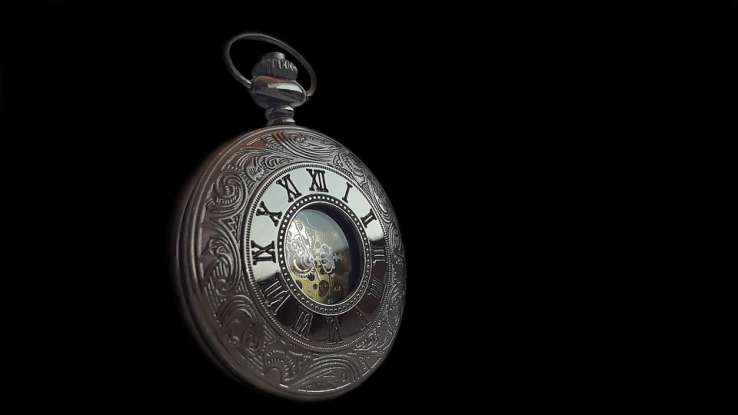1. CCVIIL

2. CLVII

3. CCLVII

4. CCLIIIIIII

The correct answer is CCLVII. Broken down, this means that C(100) + C(100) + L(50) + V(5) + II(2) gives you 257.

## 3. Lisa wants to give 4 cookies each to 7 friends. How many cookies does she need to make?1. 35

2. 24

3. 28

4. 32

The correct answer is 28. 7 x 4 = 28.

## 4. If I have 2/3 of pizza, and you have 7/3 of pizza, how many pizzas do we have together?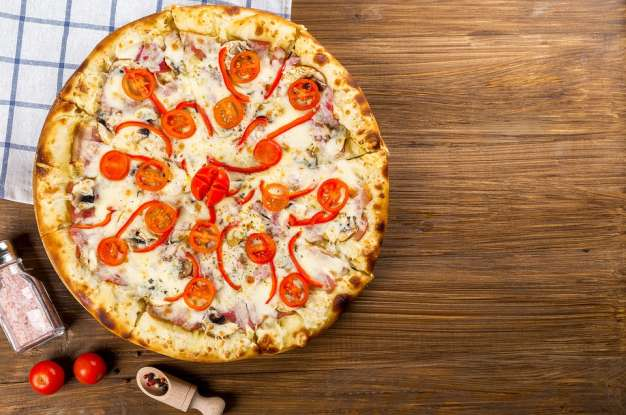1. 4

2. 3

3. 2

4. 7

The correct answer is 3. 2/3 + 7/3 = 9/3. One pizza is 3/3, so 9/3 are three pizzas.

## 5. How would you express the fraction 3/5 in decimals?1. 0.75

2. 0.55

3. 0.60

4. 0.66

The correct answer is 0.60. 1/5 is 0.20 and 0.20 x 3 = 0.60.

## 6. What times 5 gives you 125?1. 25

2. 35

3. 15

4. 20

The correct answer is 25. You can solve this by dividing 125 by 5, which is 25.

## 7. Yesterday Joe caught 23 fish, and today he's caught 39. However, 4 were too small and he returned them to the water. How many fish does he now have in total?1. 62

2. 60

3. 58

4. 57

The correct answer is 58. 23 + 39 = 62 and 62 - 4 = 58.

## 8. How many inches are in 12 feet?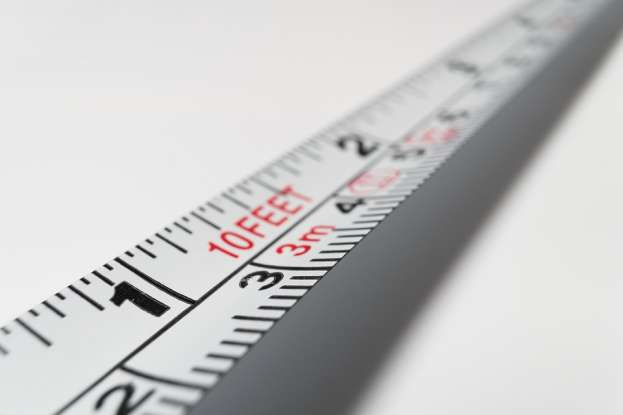1. 140

2. 152

3. 144

4. 112

The correct answer is 144. 1 foot is 12 inches, so 12 x 12 = 144.

## 9. What is the solution to 3 x 2 + 30 x (1 x 5)?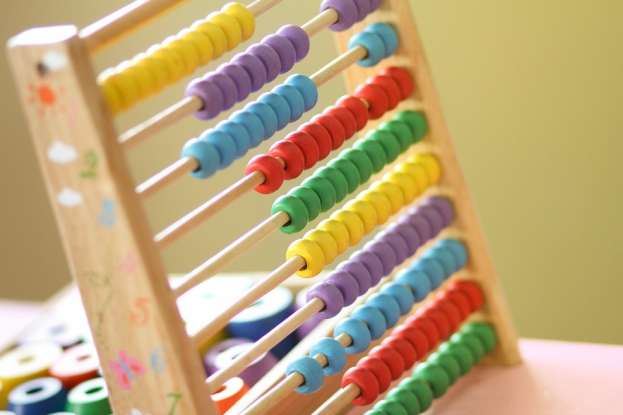1. 156

2. 330

3. 450

4. 178

The answer is 156. The equation follows this order of operations: parentheses, multiplications and divisions, then additions and subtractions.

## 10. Juan and Pablo are eating pie. Juan eats 3/4 of his pie and Pablo eats 6/8 of his own. Who has more pie left?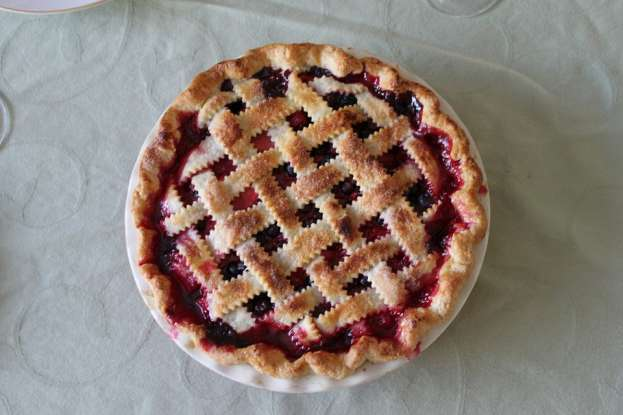1. Juan

2. Pablo

3. They have the same amount left

4. Neither has any pie left

The correct answer is that they each have the same amount of pie left. Both 3/4 and 6/8 are equal to 75% each.

## 11. Gardeners had to plant 340 trees in three days. The first day they plant 107 trees and the second day 92. How many do they have to plant the third day?1. 325

2. 135

3. 101

4. 141

The correct answer is 141. Take your total number of trees and subtract what you have the first two days to get the remaining number of trees: 340 - 107 - 92 = 141.

## 12. What kind of angle is shown here?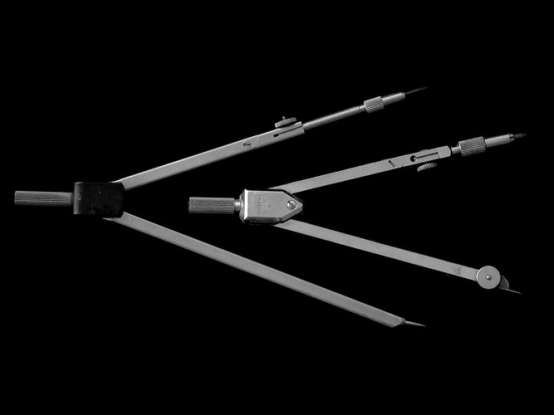1. Right

2. Acute

3. Obtuse

4. Reflex

The correct answer is acute. Acute angles are anything smaller than right angles (90 degrees).

## 13. The Roman numeral MCCLIV represents what number?1. 1254

2. 1355

3. 1255

4. 1154

The correct answer is 1254. M(1000) + CC(200) + L(50) + IV(4) = 1254.

## 14. A restaurant has 37 tables. Each table has 4 chairs. What is the maximum capacity of the restaurant?1. 138

2. 148

3. 158

4. 37

The correct answer is 148. Take the number of chairs times the number of tables to get this total: 37 x 4 = 148

## 15. What are the factors of 23?1. 1, 12, 23

2. 1, 23

3. 23

4. 1, 11, 23

The correct answer is 1, 23. Factors are the numbers by which a number can be divided (without resulting in decimals).

## 16. A building has 17 floors and 102 rooms. How many rooms are there on each floor, assuming each floor has the same number of rooms?1. 6

2. 5

3. 7

4. 4

The correct answer is 6. 102 (rooms total) divided by 17 (floors) is 6 (rooms per floor).

## 17. Which of the following is a prime number?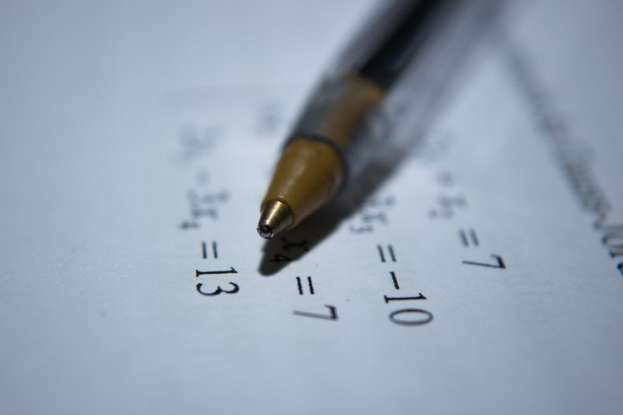1. 14

2. 25

3. 13

4. 36

The correct answer is 13. Prime numbers can only be divided by themselves and 1.

## 18. Sunil bought 89 candy bars to share among his 11 friends, but he ate 12 candy bars before meeting them. How many bars will each of his friends get now?1. 7

2. 8

3. 6

4. 5

The correct answer is 7. First, take 12 from 89 to get 77 candy bars available to share. Then divide the number of bars left (77) by the number of friends (11); this gives you 7.

## 19. A rectangle is 9 inches in length and 6 inches in width. How much is its area?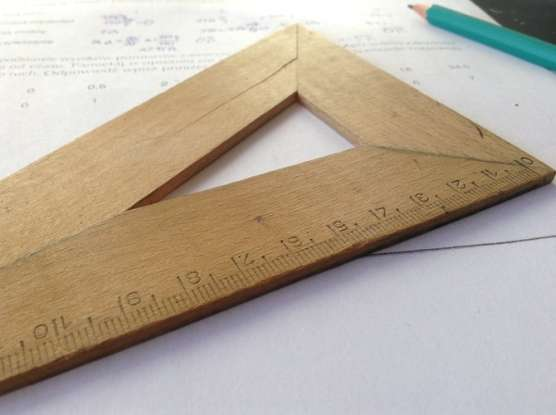1. 15 inches

2. 3 inches

3. 48 inches

4. 54 inches

The correct answer is 54 inches. To find the area of a rectangle, you have to multiply its length times its width. So, 9 in x 6 in = 54 in.

## 20. Eva had a collection of 265 bottle caps. When she moved, she lost a box with 34 caps, but then acquired 42 new ones. How many caps does she have now?1. 286

2. 269

3. 273

4. 265

The correct answer is 273. Start with the original number of caps (265) and subtract how many she lost (34), then add how many she acquired (42) to get her new total (273).

## 21. Jonah earned \$14.77 on tips yesterday, and today he made \$19.45. How much did he earn in total?1. \$34.22

2. \$33.42

3. \$33.32

4. \$34.12

The correct answer is \$34.22. \$14.77 + \$19.45 = \$34.22.

## 22. How many meters are there in 345 kilometers?1. 3450 meters

2. 0.345 meters

3. 345,000 meters

4. 34,500 meters

The correct answer is 345,000 meters. One kilometer is equal to 1000 meters, so 345 m x 1000 = 345,000 km.

## 23. Lina has made 3 kg of strawberry jam and she wants to put it in jars that can hold 200 g of jam each. How many jars does she need?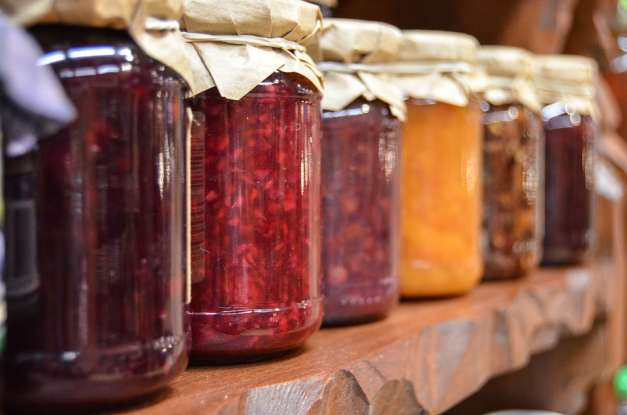1. 15

2. 150

3. 1500

4. 1.5

The correct answer is 15. One kilogram equals 1000 grams, so 3 x 1000 gives you 3000 g of jam total. Divide this by how much each jar can hold (200 g) to get the correct number of jars (15).

## 24. If the radius of a circle is 4, how much is its diameter?1. 2

2. 4.5

3. 8

4. 3.14

The correct answer is 8. The radius is the distance between the center and the edge of a circle. The diameter goes through the center of the circle, making the diameter worth two radii. So, 4 x 2 = 8.

## 25. What is the name of the shape shown here?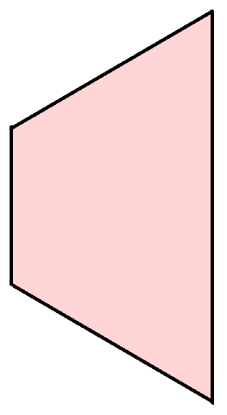1. Pentagon

2. Hexagon

3. Triangle

4. Trapezoid

The correct answer is a trapezoid, which is a quadrilateral with at least one pair of parallel sides.

## 26. If now it's 3:45 AM, what time will it be in 2 hours and 20 minutes?1. 5:10 AM

2. 6:05 AM

3. 6:15 AM

4. 5:55 AM

The correct answer is 6:05 AM. Hours are measured in 60 minutes. So 20 minutes past 3:45 AM gives us 4:05 AM. Two hours past this is 6:05 AM.

## 27. Paul can type 2345 words per hour. How many words can he type in 6 hours?1. 15,010

2. 14,185

3. 15,060

4. 14,070

The correct answer is 14,070. 2345 (words) x 6 (hours) = 14,070 (words total).

## 28. Sam works Monday to Friday from 10:00 AM to 6:00 PM. Monday to Thursday he earns \$5 per hour, but Friday he earns \$6 per hour. How much does he earn in a week?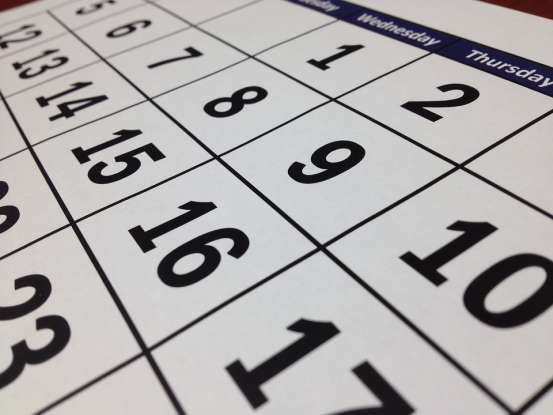1. 318

2. 256

3. 208

4. 119

The correct answer is 208. Monday to Thursday he earns \$40/day (\$5 x 8 hr = \$40/day). This happens for 4 days, so he has \$160 (\$40/day x 4 days = \$160). On Friday, he makes \$48 (\$6 x 8 hr = \$48/day). \$160 + \$48 = \$208.

## 29. 3 kilograms of apples cost \$9 and 5 kilograms cost \$15. How much is a kilogram of apples worth?1. \$2

2. \$5

3. \$4

4. \$3

The correct answer is \$3. It doesn't matter if you buy 3 kg or 5 kg, the price is the same: 9 / 3 = 3 and 15 / 5 = 3.

## 30. 1, 4, 2, 8, 3, 12: What are the next two numbers in this series?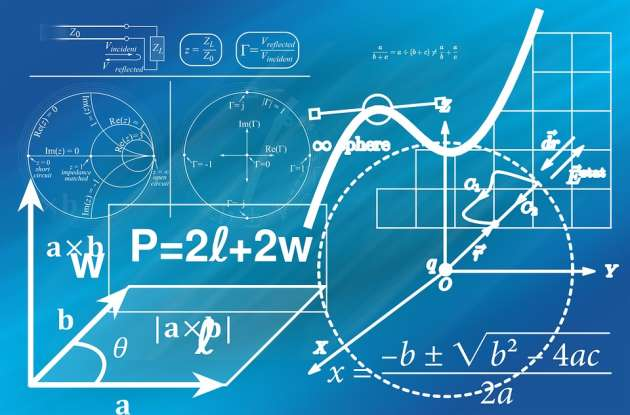1. 4, 15

2. 5, 20

3. 4, 16

4. 5, 15

The correct answer is 4, 16. The odd numbers progress in a +1 chronological order, so logically the seventh number in the series should be 4, since the fifth number was 3 (3 + 1 = 4). The even numbers in this series are the preceding number multiplied by 4. So the eighth number in the series should be 16, since the preceding number is 4 (4 x 4 = 16).

## 31. The farmers harvested 25 kg of peaches, 15 kg of plums, 24 kg of potatoes, and 30 kg of cherries. How many kilograms of fruit do they have in total?1. 94

2. 70

3. 84

4. 40

The correct answer is 70 kg total, solved by the equation 25 kg (peaches) + 15 kg (plums) + 30 kg (cherries) = 70 kg of fruit. Potatoes are not fruits!

## 32. A textile factory uses 5 yards of fabric to make a dress. How many dresses can be made with 255 yards of fabric?1. 65

2. 255

3. 51

4. 55

The correct answer is 51. 255 (total yards) divided by 5 (yards needed to make 1 dress) is 51 (dresses possible).

## 33. How many ounces (oz) are there in 25 pounds (lb)?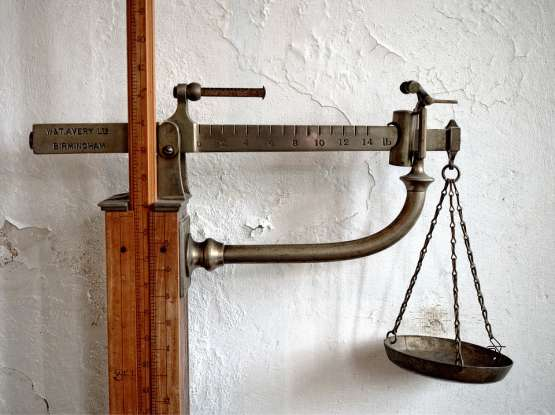1. 400

2. 100

3. 350

4. 275

The correct answer is 400. 1 lb equals 16 oz, so 25 x 16 = 400.

## 34. John and Diane own a field. John owns 12/16 of the field, whereas Diane owns 2/8. Who owns 75% of the field?1. Diane

2. John

3. Neither

4. Both

The correct answer is John. 12/16 equals 0.75 or 75%.

## 35. Alex's house is 270 miles away from London while Paula's is 270 kilometers away. Which house is closest to London?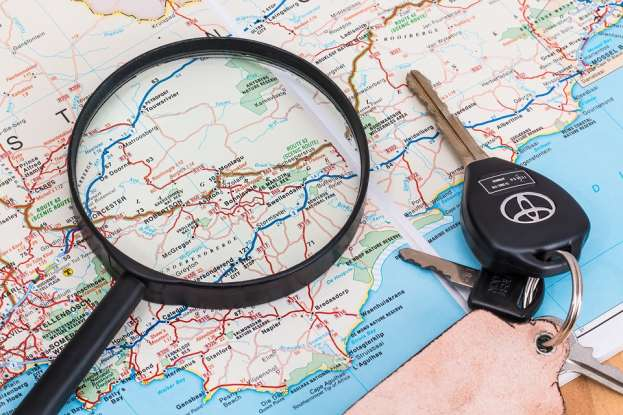1. Alex's house

2. Paula's house

3. Equal distance

4. Cannot tell with the provided information

The correct answer is Paula's house. One mile roughly equals 1.6 kilometers. So, divide 270 mi by 1.6 to get roughly 168 mi as the distance for Paula's house, which is closer to London.

## 36. Four friends go to dinner and decide to split the bill evenly. The total is \$48.88. How much will each of them pay?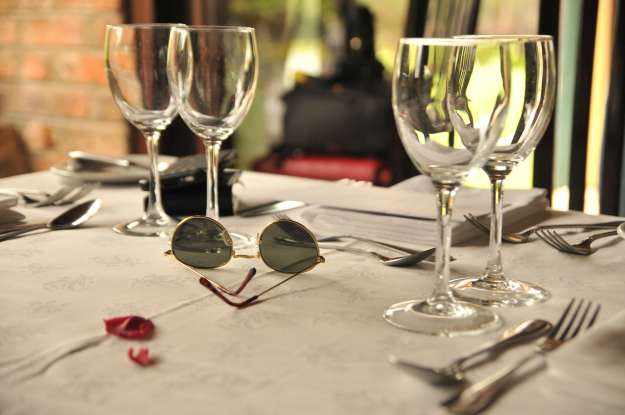1. \$13.22

2. \$12.22

3. \$14.22

4. \$15.22

The correct answer is \$12.22. 48.88 divided by 4 is 12.22.

## 37. Kabir had 155 kg of fruit when he opened his shop in the morning. He sold 10 kg of apples, 15 kg of pears, and 50 kg of oranges. How many kilograms of fruit does he have left?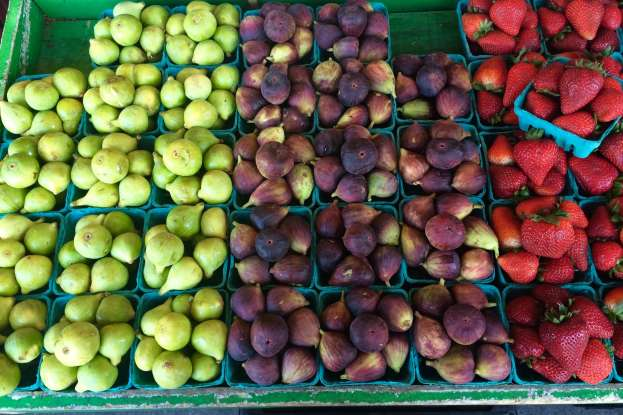1. 80

2. 100

3. 95

4. 85

The correct answer is 80. There are two ways of putting it: 155 - 10 - 15 - 50 = 80 or 155 - (10 + 15 + 50) = 80. Essentially, take the beginning amount of fruit and subtract the amount sold to get what is left.

## You Did It!

Great job! You got 100% because you're a bot and I have all the asnwered laid out for you!You're a math genius!

Amazing job! Why not share your score to let your friends know they need to invite you over to tutor their kids?